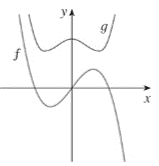# Whether the given functions graph is even, odd, or neither.### Single Variable Calculus: Concepts...

4th Edition
James Stewart
Publisher: Cengage Learning
ISBN: 9781337687805### Single Variable Calculus: Concepts...

4th Edition
James Stewart
Publisher: Cengage Learning
ISBN: 9781337687805

#### Solutions

Chapter 1.1, Problem 63E
To determine

## To find: Whether the given functions graph is even, odd, or neither.

Expert Solution

### Explanation of Solution

Given information:

The given graph is,The function is said to be even function if it satisfies f(x)=f(x) for all values of x . Here, the function g satisfies the condition that is g(x)=g(x) . Hence, function g is the even function.

The function is said to be odd function if it satisfies f(x)=f(x) for all values of x . Here, the function f satisfies the condition that is f(x)=f(x) . Hence, function f is the odd function.

### Have a homework question?

Subscribe to bartleby learn! Ask subject matter experts 30 homework questions each month. Plus, you’ll have access to millions of step-by-step textbook answers!